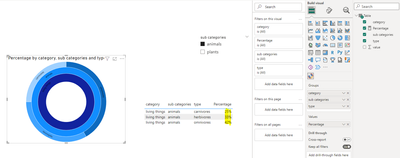cancel
Showing results for
Did you mean:Frequent Visitor

## Sunburst: displaying the percentage based on selected category

I have a sunburst chart with a category and two sub categories. I want my sunburst chart to show the percentage based on the selected category/subcategory. For eg: I have two subcategories 'plants' and 'animals' with 'living things' as my main category. So when I choose 'animals' sub category,  I want my sunburst to show the percent of carnivores, herbivores and omnivores out of 100, so I do not include the percent of plants in the calculation.

Thank you so much in advance!

1 ACCEPTED SOLUTIONCommunity Support

According to your description, here are my steps you can follow as a solution.

(1) This is my test data.(2) We can create a measure.

``````Percentage =
var _sub=SELECTEDVALUE('Table'[sub categories])
var _a=CALCULATE(SUM('Table'[value]),FILTER(ALL('Table'),'Table'[sub categories]=_sub && 'Table'[type]=MAX('Table'[type])))
var _b=CALCULATE(SUM('Table'[value]),FILTER(ALL('Table'),'Table'[sub categories]=_sub))
return DIVIDE(_a,_b,0)``````

(3) Then the result is as follows.If the above one can't help you get the desired result, please provide some sample data in your tables (exclude sensitive data) with Text format and your expected result with backend logic and special examples. It is better if you can share a simplified pbix file. Thank you.

Best Regards,

Neeko Tang

If this post  helps, then please consider Accept it as the solution  to help the other members find it more quickly.Community Support

According to your description, here are my steps you can follow as a solution.

(1) This is my test data.(2) We can create a measure.

``````Percentage =
var _sub=SELECTEDVALUE('Table'[sub categories])
var _a=CALCULATE(SUM('Table'[value]),FILTER(ALL('Table'),'Table'[sub categories]=_sub && 'Table'[type]=MAX('Table'[type])))
var _b=CALCULATE(SUM('Table'[value]),FILTER(ALL('Table'),'Table'[sub categories]=_sub))
return DIVIDE(_a,_b,0)``````

(3) Then the result is as follows.If the above one can't help you get the desired result, please provide some sample data in your tables (exclude sensitive data) with Text format and your expected result with backend logic and special examples. It is better if you can share a simplified pbix file. Thank you.

Best Regards,

Neeko Tang

If this post  helps, then please consider Accept it as the solution  to help the other members find it more quickly.